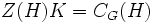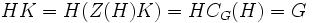# Hall and central factor implies direct factor

This article gives a proof/explanation of the equivalence of multiple definitions for the term Hall direct factor
View a complete list of pages giving proofs of equivalence of definitions

## Statement

Any Hall subgroup of a finite group that is a central factor of the group, is also a direct factor.

## Facts used

1. Second isomorphism theorem
2. Normal Hall implies permutably complemented (this is the first half of the Schur-Zassenhaus theorem)

## Proof

Given: A finite group$G$, a Hall subgroup$H$ such that$HC_G(H) = G$.

To prove:$H$ is a direct factor of$G$.

Proof: Consider the subgroup$C_G(H)$. The subgroup$Z(H) = H \cap C_G(H)$ is central in$C_G(H)$. Also, by the second isomorphism theorem,$C_G(H)/(H \cap C_G(H)) \cong HC_G(H)/H = G/H$, so$Z(H)$ is a Hall subgroup of$C_G(H)$.

Thus,$Z(H)$ is a central Hall subgroup of$C_G(H)$.

In particular$Z(H)$ is normal Hall in$C_G(H)$, so it possesses a complement, say$K$, in$C_G(H)$. Clearly,$H$ and$K$ permute element-wise, because$K \le C_G(H)$, and$H \cap K$ is trivial, because$K \cap (H \cap C_G(H))$ is trivial by construction. Finally,$Z(H)K = C_G(H)$ by construction, so$HK = H(Z(H)K) = HC_G(H) = G$, and so,$H$ and$K$ are complements.

Thus:$H$ and$K$ commute element-wise, generate the whole group, and intersect trivially, making$G$ an internal direct product of$H$ and$K$, and thus making$H$ a direct factor of$G$.